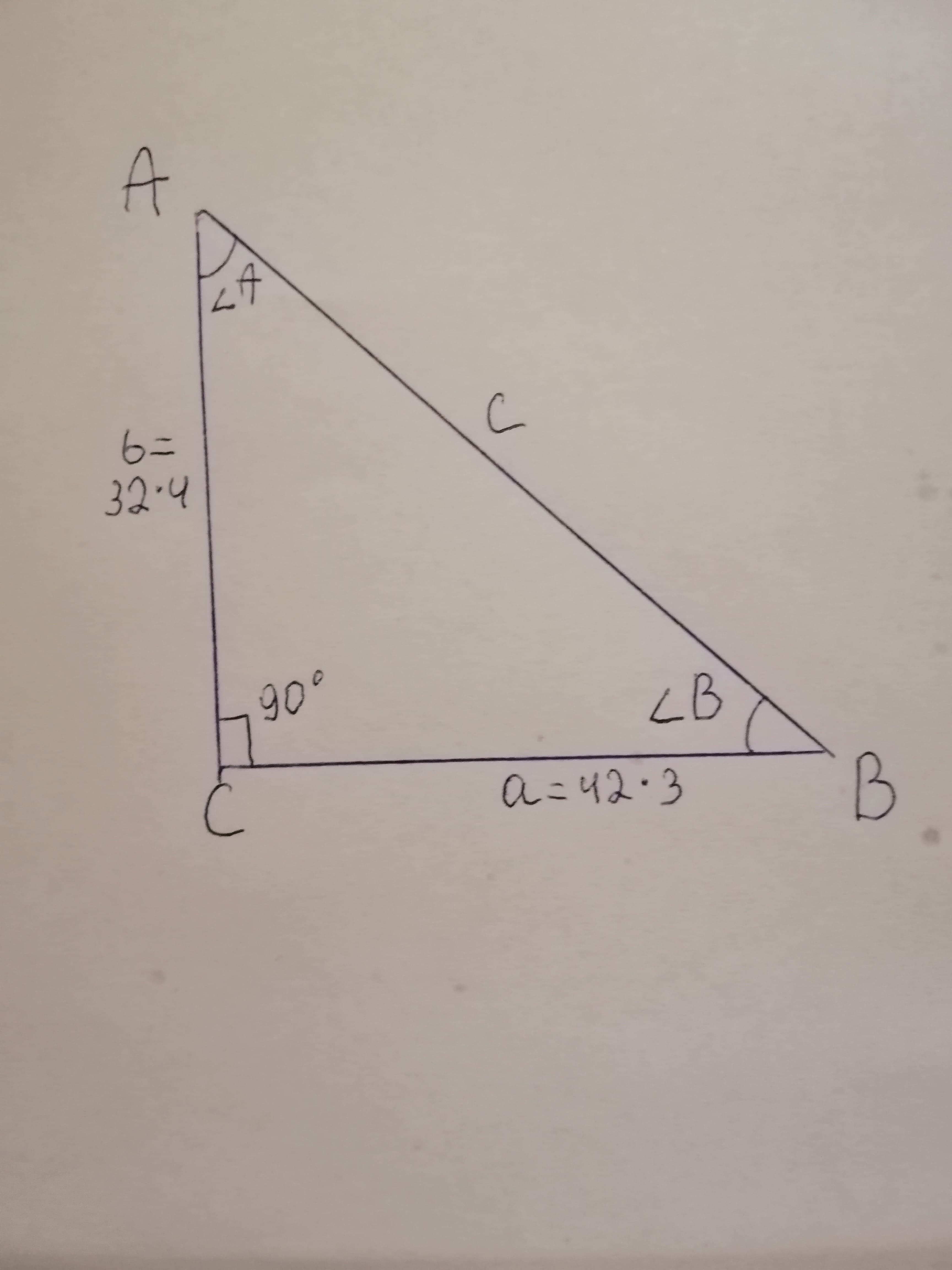# Refer to right triangle ABC with C = 90^{circ}. Begin each problem by drawing a picture of the triangle with both the given and asked­for information labeled appropriately. Also, write your answers for angles in decimal degrees. If a = 42.3 inches and b = 32.4 inches, find BGlobokim8 2021-01-15 Answered
Refer to right triangle ABC with $C={90}^{\circ }.$ Begin each problem by drawing a picture of the triangle with both the given and asked­for information labeled appropriately.
If inches, find B
You can still ask an expert for help

• Questions are typically answered in as fast as 30 minutes

Solve your problem for the price of one coffee

• Math expert for every subject
• Pay only if we can solve itElberte

Data analysis
Given right angled triangle at C for a triangle
And $a=42.3$ inches
$b=32.4$ inches
To find angle at B.
The triangle with above data is as followsSolution
Since the give triangle is right angled,
Applying Pythagoras theorem,

Hence the length of $AB=c=53.28$ inches
(Rounding to two decimals)
By sine law,

$=AC/AB$
$=b/c$
$=32.4/53.28$

Hence the angle at B is ${37.24}^{\circ }$
(Rounding to two decimals)